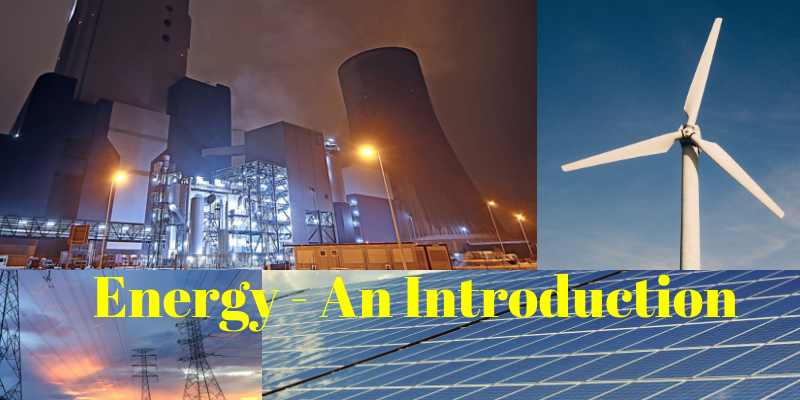Basics

# The basics of Energy

We have talked a lot about energy efficiency, energy storage and energy generation. We have energy in all the terms we have discussed so far. To understand all these better, the term energy has to be understood in a better way. This post aims to define energy in an easy and simple way.

Energy

Energy, in simple terms, is the ability to do work either physically or mentally. The law of conservation of energy states that the energy can be neither created nor destroyed, but can be transformed from one form to another from with some loss. Energy is basically classified into potential energy and kinetic energy. Potential energy is the energy possessed by a body by the virtue of its position in relative to other objects. Kinetic Energy is the energy possessed by the body due to its motion.

Electric Energy

The electrical energy is the ability to make electrical and electronic equipment work. It is the combination of electric current and electric potential. Micheal Faraday described electric energy as the movement of conductor between poles of magnet. Electric energy has wide need. It is used everywhere in domestic, commercial, industrial applications. One cannot imagine a world without electrical energy today.

Electricity

Electricity is the flow of electrons around a circuit. Electricity can be two types. Static Electricity and Current Electricity. Static electricity is obtained by rubbing two neutral bodies, while current electricity is gained by the flow of current.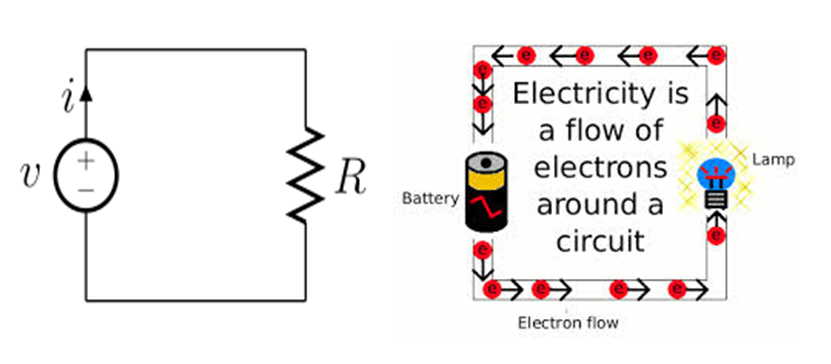Electric Current

The flow of charge is called electric current. These charges are positive and negative (electron). Similar to a magnet, like charges repel and opposite charges attract. While in strict terms, current has no direction, the positive side is immovable. The direction of electron is opposite to that of electron.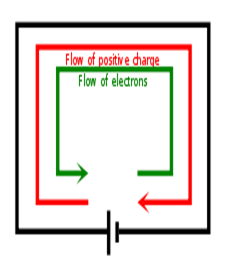Types of Current

There are two types of current viz., Direct Current and Indirect Current. The Direct Current flows in one direction while Alternating current as the name suggests flows in alternate direction.Electric Potential

Electric potential is the potential energy of a charged particle. It makes the flow of charge possible from a higher potential to lower potential.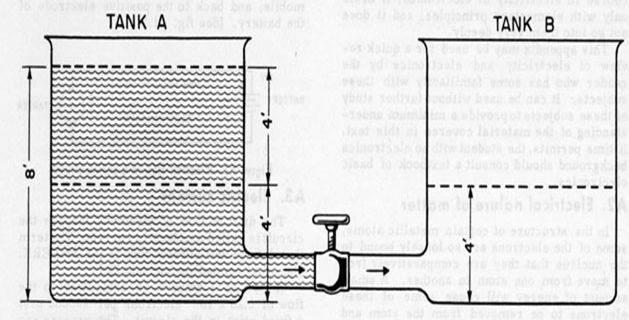Watt

Watt is the unit of power. It is the work done by one ampere of electric current with one volt of electric potential. It is mentioned as W. Thousand watt is equal to 1 Kilo Watt (KW). 1000 KW is equal to 1 Mega Watt (1 MW) and 1000 MW is equal to 1 GW.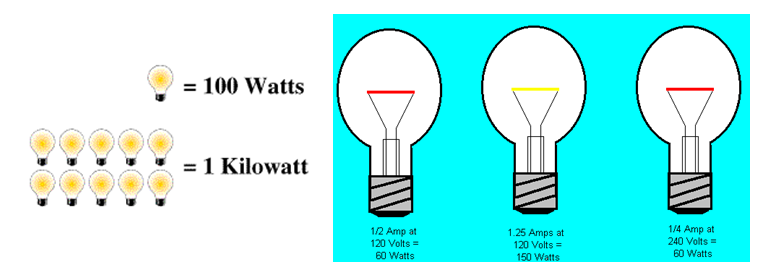Kilowatt Hour

Kilo Watt hour is the unit of energy. One Kilo Watt hour is the energy consumed by a 1000W electric equipment in one hour. It is calculated by multiplying the watt a equipment with total hours of usage and dividing by 1000. For example, a 100W Street light that burns for 12 hours consume 1.2 KWh. It is obtained by (100 x 12)/1000 = 1.2 KWh.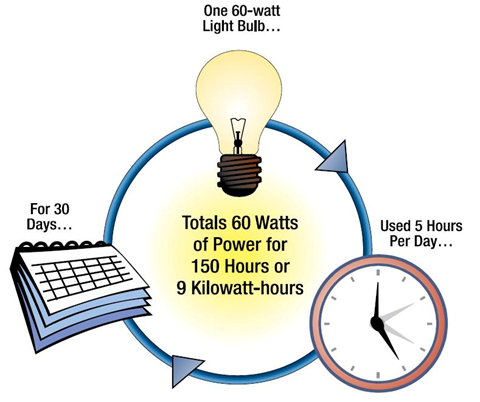Sources of Electrical Energy

Electrical energy can be obtained from renewable and non-renewable sources of energy. Non-renewable source of energy will get replenished in many lifetimes. These sources include Coal, Nuclear Power, Natural Gas, Petroleum, and Furnace Oil. These are formed over several hundreds of years. While it is easy to extract and store these, it is very harmful to burn them to produce energy. Renewable energy is from natural resources that are replenished in a lifetime. These include solar energy, wind energy, bio mass energy, geo thermal energy, hydel energy, etc.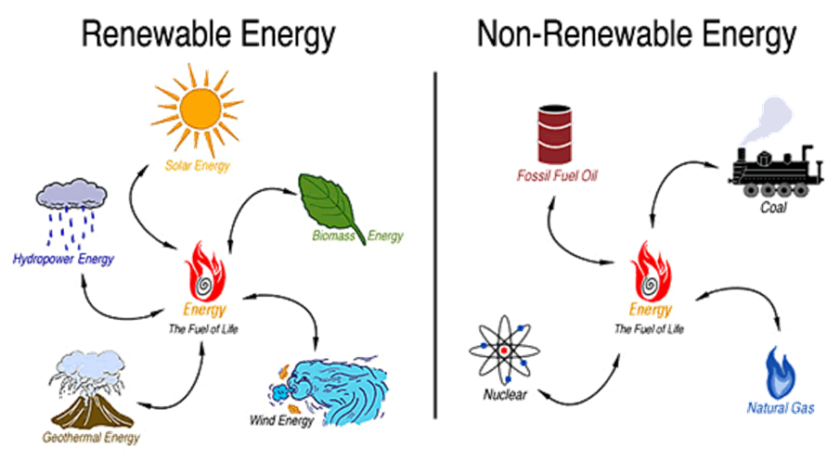Call us